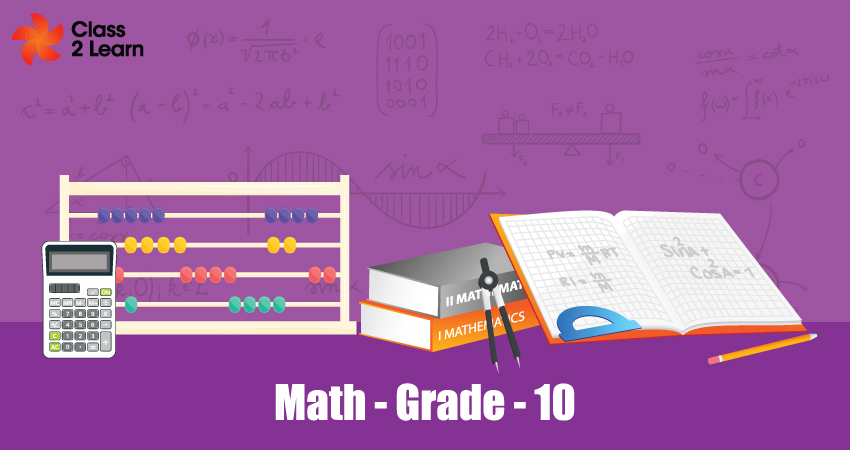# MathematicsMonthly One-time
Price
\$ 10

Per month

#### Overview

Mathematics is the science that deals with the logic of shape, quantity and arrangement. Math is all around us, in everything we do. It is the building block for everything in our daily lives. It is an area of knowledge

#### Polynomials

This chapter guides the student to develop the skills and get the deep knowledge about polynomials-Introduction, geometry meaning of zeros of polynomials, relationship between zeros and the coefficient of polynomials and division algorithm of polynomials.  Students will be expertise in learning through these concepts.

This Chapter guides the student to develop the skills and  get deep  knowledge about quadratic equations, how to find the nature of roots, methods of solving quadratic equations, Solving by completing squares, Solving by factorization. Students will be expertise in learning through these concepts.

#### Arithmetic Progressions

This Chapter guides the student to develop the skills and  get deep  knowledge about arithmetic progression, Terms involving arithmetic progression-First term and Common difference,nth term of the A.P,knowing general term of arithmetic progression, sum of first n terms of A.P,Applications of A.P. Students will be expertise in learning through these concepts.

#### Triangles

This Chapter guides the student to develop the skills and  get deep  knowledge about triangles-Similar triangles and congruent triangles. Basic proportionality theorem, criteria of similar triangles and Pythagorean theorem. Students will be expertise in learning through these concepts.

#### Geometry

This Chapter guides the student to develop the skills and  get deep  knowledge about meaning of tangents, tangents to the circle, tangents from a point on circle, Constructions of tangents to the circle, construction of similar triangles. Students will be expertise in learning through these concepts.

#### Coordinate Geometry

This Chapter guides the student to develop the skills and  get deep  knowledge about coordinate geometry and graph of linear equations, area of triangle, distance formula. Students will be expertise in learning through these concepts

#### Mensuration

This Chapter guides the student to develop the skills and  get deep  knowledge about the area of the combination of plane figures, area and perimeter of circle, Sector and segments of the circle, surface area and volume of a combination of solids. Students will be expertise in learning through these concepts.

#### Statistics and Probability

This chapter guides the student to develop the skills and get deep knowledge about statistics-Primary data,  and secondary data, measures of central tendency - Mean, Median and mode of grouped and ungrouped data, Graphical representation of cumulative frequency distribution, probability- theoretical probability and experimental probability, Conditional probability and its properties.

#### Real Numbers

This chapter guides the student to develop the skills and get the deep knowledge about decimal expansion of real numbers, terminating and non-terminating decimals, Euclid’s division lemma, fundamental theorem of arithmetic. And also student will learn about irrational numbers, expressing irrational numbers on the number line and solve the problems based on it.

#### Trigonometry

This Chapter guides the student to develop the skills and get deep knowledge about trigonometric identities, trigonometric ratios of complementary angles, trigonometric ratios of specific angles, some applications of trigonometry. Students will be expertise in learning through these concepts.

#### Benefits

##### Included in this plan
• e-books
• Test Series & Analysis
• Assignments & Notes
• Hands on Activity
Free Trial Class

Take advantage of our free trial!